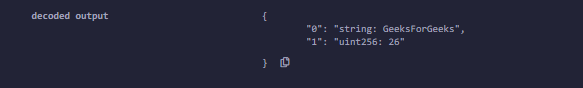# Solidity – Basics of Interface

• Last Updated : 11 May, 2022

Interfaces are the same as abstract contracts created by using an interface keyword, also known as a pure abstract contract. Interfaces do not have any definition or any state variables, constructors, or any function with implementation, they only contain function declarations i.e. functions in interfaces do not have any statements. Functions of Interface can be only of type external. They can inherit from other interfaces, but they can’t inherit from other contracts. An interface can have enum, structs which can be accessed using interface name dot notation.

Example: In the below example, the contract thisContract implements an interface InterfaceExample and implements all the interface functions.

## Solidity

 `// Solidity program to ``// demonstrate the working ``// of the interface`` ` `pragma solidity 0.4.19;`` ` `// A simple interface``interface InterfaceExample{`` ` `    ``// Functions having only ``    ``// declaration not definition``    ``function getStr(``    ``) ``public` `view returns(string memory);``    ``function setValue(``      ``uint _num1, uint _num2) ``public``;``    ``function add(``    ``) ``public` `view returns(uint);``}`` ` `// Contract that implements interface``contract` `thisContract is InterfaceExample{`` ` `    ``// Private variables``    ``uint ``private` `num1;``    ``uint ``private` `num2;`` ` `    ``// Function definitions of functions ``    ``// declared inside an interface``    ``function getStr(``    ``) ``public` `view returns(string memory){``        ``return` `"GeeksForGeeks"``;``    ``}``     ` `     ``// Function to set the values ``    ``// of the private variables``    ``function setValue(``      ``uint _num1, uint _num2) ``public``{``        ``num1 = _num1;``        ``num2 = _num2;``    ``}``     ` `    ``// Function to add 2 numbers ``    ``function add(``    ``) ``public` `view returns(uint){``        ``return` `num1 + num2;``    ``}``     ` `}`` ` `contract` `call{``     ` `    ``//Creating an object``    ``InterfaceExample obj;`` ` `    ``function call() ``public``{``        ``obj = ``new` `thisContract();``    ``}``     ` `    ``// Function to print string ``    ``// value and the sum value``    ``function getValue(``    ``) ``public` `returns(uint){``        ``obj.getStr;``        ``obj.setValue(10, 16);``        ``return` `obj.add();``    ``}``}`

Output :My Personal Notes arrow_drop_up